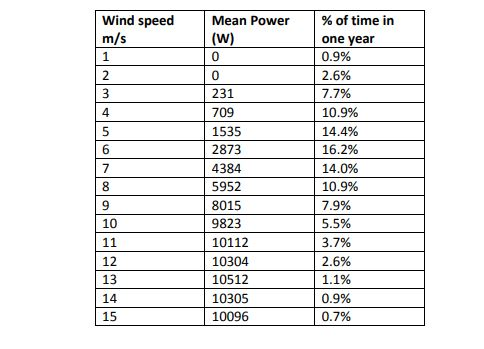Wind Energy

EXERCICE: WIND ENERGY

Let us consider a wind turbine with a rotor diameter of 8m and 3 blades.The performance measurements according to the wind speed are given in the table below:1) The manufacturer displays a rated power of 10 KW for 10m/s. is it exact?

2) Deduce the efficiency coefficient Cp of the wind turbine at 10m/s. The density of air ρ = 1.225kg /m3.

3) Calculate the annual operating time D: The annual operating time is defined as the quotient of the energy bought back during a year (365 days) of operation of the installation by the maximum active power of the same installation (10 kW).

4) The investment cost of this wind turbine is 26.500 euros. operation and maintenance costs are estimated at 500 euros / year. In order to evaluate the return on investment and given EDF’s electricity purchase price as defined below. calculate the payback period PBT of the installation (time for which the wind turbine is reporting as much as it has cost).CORRECTION:

1) According to the mean power is 9823 W. It is a mean value near 10000 W. Therefore. we can admit the rated power displayed by the manufacturer. It is exact.

2) Cp=Péol/0.5×ρ×π×R2×V3=9823/0.5×1.225×π×42×103=0.31

3) Cp=Annual operating time D=Total energy per year produced/maximum active power 10kWD=Total energy per year produced/maximum active power 10kW=36242kWh/10kW=3624h

4) Expected revenues for the first 10 years:

R= 36242 × 0.082 − 500=2472euros/year

Expected revenues for the following 5 years:

R= 36242 × 0.028 − 500=515euros/year

Depreciation period: PBT= 13.5 years as ∶ 26500  ≅ 10 years × 2472€ + 3.5 years × 515 € = 13.5